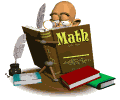•Math Curriculum
• Data, Graphs, Facts Review
• Place Value and Time
• Adding Whole Numbers and Money
• Subtracting Whole Numbers and Money
• Multiplication Concepts and Facts
• Division Concepts and Facts
• Using Geometry
• Fractions
• Standard Linear Measurement
• Decimals
• Metric Linear Measurement
• Measurement and ProbabilityKnowing math facts makes learning new concepts easier!
It is very important for the children to practice
their math facts on a regular basis!